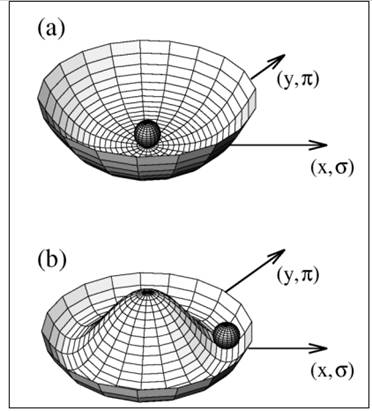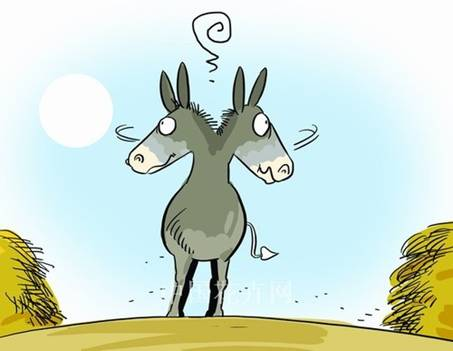Blockvorlesung (Block lecture)

“Effective hadronic theories and models ”

Time:                   Mo. 18/8/2014 – Fr. 22/8/2014. Time: 12:00 (c.t.) -15:00.

Room:                 02.114 (Aquarium room).

Dozent:                Francesco Giacosa

Email:                 giacosa@th.physik.uni-frankfurt.de

`This is a series of introductory lectures on QCD and chiral theories for master and PhD students. It will be in English. `
` `
`The topics are: Groups, QCD Lagrangian and its symmetries (with special attention to dilatation symmetry and chiral symmetry), `
`large Nc, multiplets of quarks, glueballs, effective approaches of QCD, such as chiral models and chiral perturbation theory.`
` `
` `
`Detailed program (preliminary): `
` `
`Monday 18/8:     Introductory considerations on QCD: pdf. `
`                             Groups U(N) and SU(N): pdf`
`                            Yang-Milss Lagrangian. Dilatation symmetry and its breaking. Dilaton field and the dilaton potential: pdf. `
`                        `
`Tuesday 19/8:      How to read the PDG: composition of a quark and an antiquark in a nonrelativistic language: spin S, angular momentum L,   `
`                             and total spin J; Parity and Charge Conjugation. Phenomenology of mesons: pdf. `
` `
`Wednesday 20/8: Composition of a quark and an antiquark in a relativistic language: Quark-antiquark bilinears. P and C.`
`                             Quark-antiquark multiplets for Nf=2 and Nf=3: pdf.`
`                              Chiral transformations: pdf. `
`                   `
`Thursday 21/8:    Spontanoeus symmetry breaking. Construction of an effective model of QCD (without and with vector d.o.f.). Decays of `
`                             mesons.: pdf. `
`                             Results of the extended Linear Sigma Model (with vector mesons): pdf. `
`                             Introduction to chiral perturbation theory: pdf. `
`                             `
`Friday 22/8:        Condensation: what is that? And what is its fluctuation? pdf`
`                             Large-Nc: short introduction for mesons anf glueballs: pdf.`
`                             Baryons: the origin of the nucleon mass. Implementation in effective models: pdf and pdf.`
`                             Tetraquarks: pdf. `
` `
`Literature (obviously only approximate…these are only some of the texts I have used…):`
` `
` `
`QCD (in general):`
`U. Mosel, Fields, symmetries, and quarks, Berlin, Germany: Springer (1999) 310 p`
`A. W. Thomas and W. Weise, The Structure of the Nucleon, Berlin, Germany: Wiley-VCH (2001) 389 p`
` `
`Meson phenomenology:`
```E. Klempt, Glueballs, hybrids, pentaquarks: Introduction to hadron spectroscopy and review of selected topics,
hep-ph/0404270.```
```E. Klempt and A. Zaitsev, Glueballs, Hybrids, Multiquarks. Experimental facts versus QCD inspired concepts,
Phys. Rept. 454 (2007) 1 [arXiv:0708.4016 [hep-ph]].```
` `
`Linear sigma Model and tis extensions:`
`My habilitation: http://th.physik.uni-frankfurt.de/~giacosa/index-Dateien/habilitation.pdf `
`ann all the theses of the chiral group in Ffm, see: http://th.physik.uni-frankfurt.de/~giacosa/chiralgroup.html `
` `
`ChPT: `
`S.Scherer, Introduction to chiral perturbation theory,`
`Adv. Nucl. Phys  27 (2003) 277, [hep-ph/0210398].`
```J.Gasser, Light quark dynamics,
Lect. Notes Phys. 629 (2004) 1[hep-ph/0312367].```
` `
`Largen-Nc:`
`E.  Witten, Baryons in the 1/n Expansion, Nucl. Phys. B 160 (1979) 57.`
`R. F. Lebed, Phenomenology of large N(c) QCD,Czech. J. Phys. 49 (1999) 1273 [nucl-th/9810080].`
` `
```

```
` `
` `
` `
` `
```
```
` `
` `
` `